### Discover more content...

Enter some keywords in the search box above, we will do our best to offer you relevant results.

### We're sorry!

We couldn't find any results for your search. Please try again with another keywords.

## 一. Clustering

### 1. 定义

$$\left\{ (x^{(1)},y^{(1)}),(x^{(2)},y^{(2)}),\cdots,(x^{(m)},y^{(m)}) \right\}$$

$$\left\{ (x^{(1)}),(x^{(2)}),(x^{(3)}),\cdots,(x^{(m)}) \right\}$$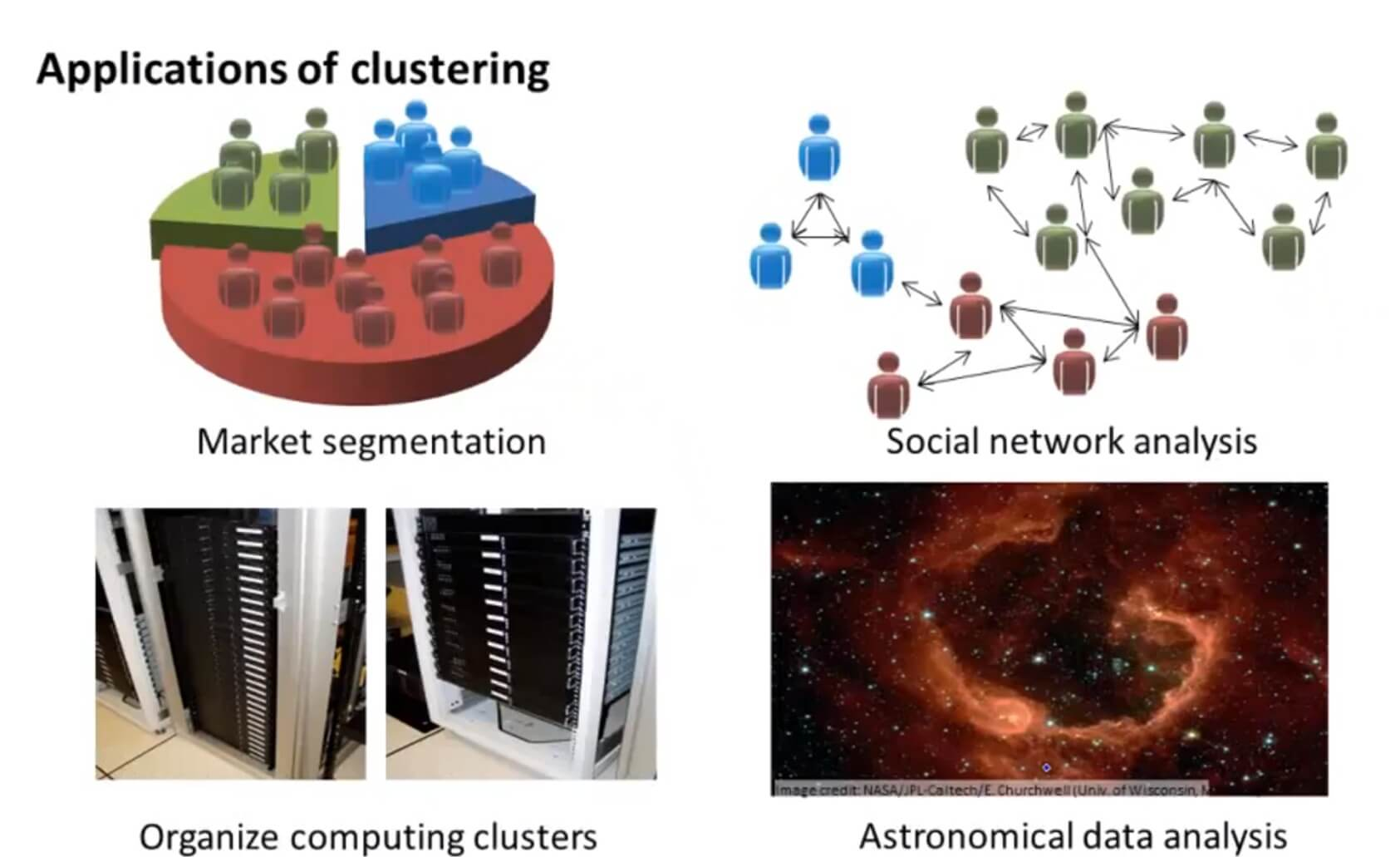### 2. K-Means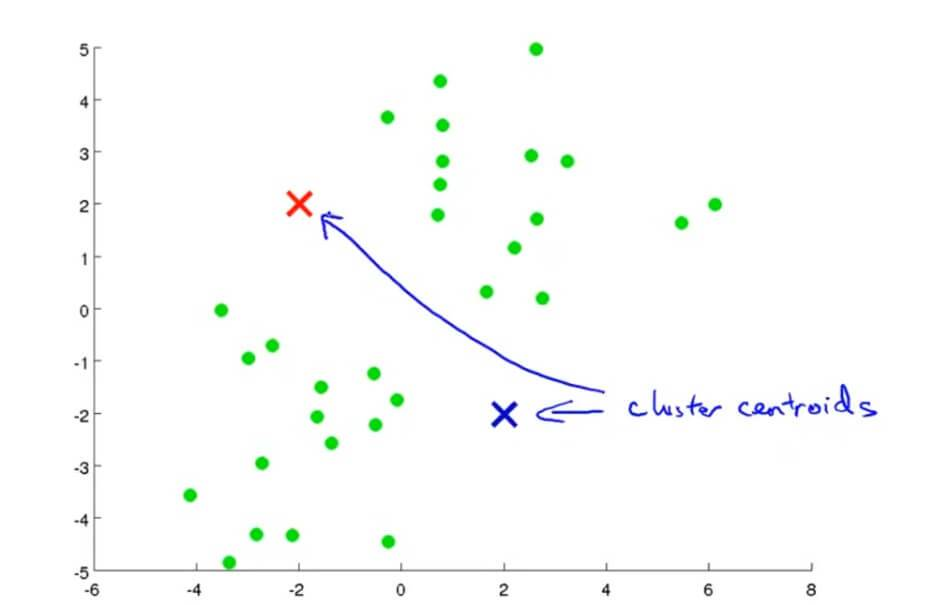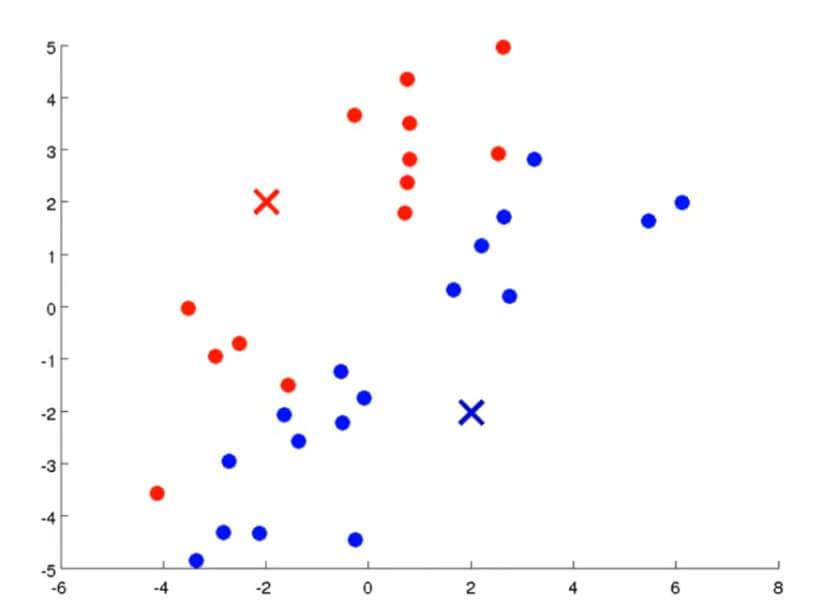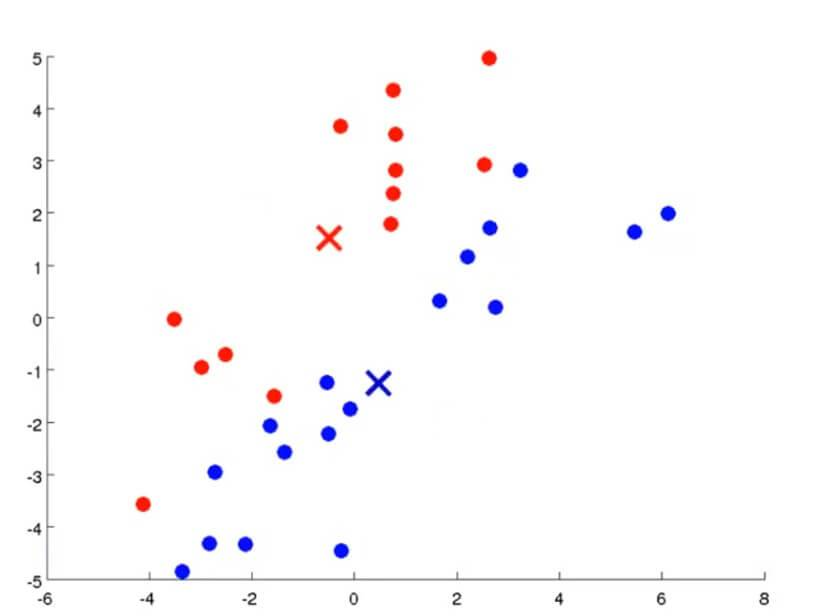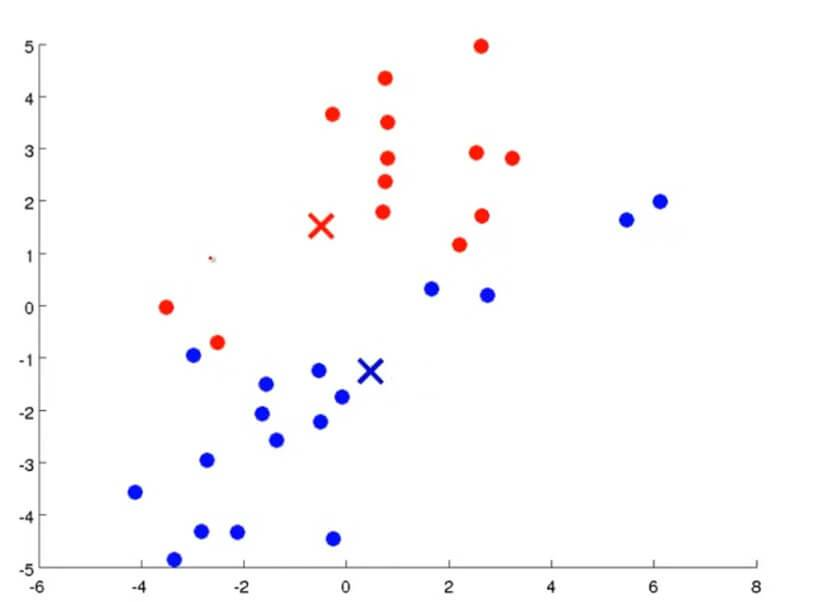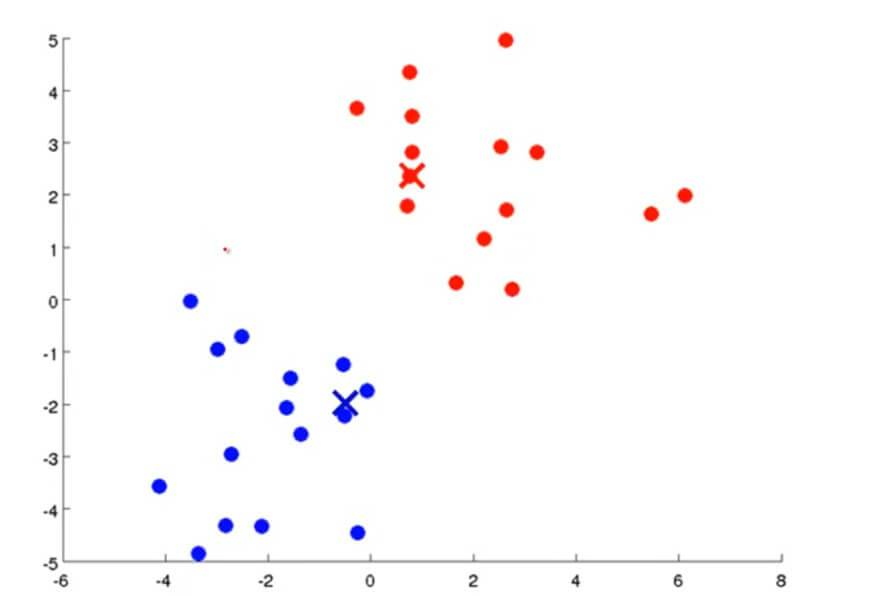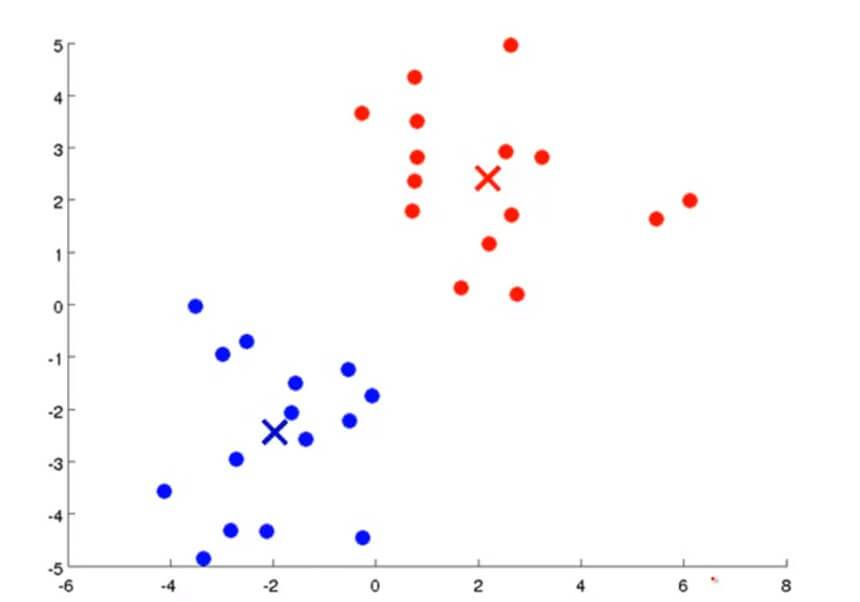K均值聚类算法有两个输入：一个是参数K，也就是你想从数据中聚类出簇的个数。另一个就是只有x没有y的训练集。

### 3. 优化

$\mu^{(i)}_c$=样本 $x^{(i)}$ 被分配到的聚类中心

$$J(c^{(1)},c^{(2)},\cdots,c^{(m)};\mu_1,\mu_2,\cdots,\mu_k)=\frac{1}{m}\sum_{i=1}^m\left \| x^{(i)}-\mu_c(i) \right \|^2$$

J 也被称为失真代价函数(Distortion Cost Function),可以在调试K均值聚类计算的时候可以看其是否收敛来判断算法是否正常工作。

1. 样本分配时：

1. 中心移动时：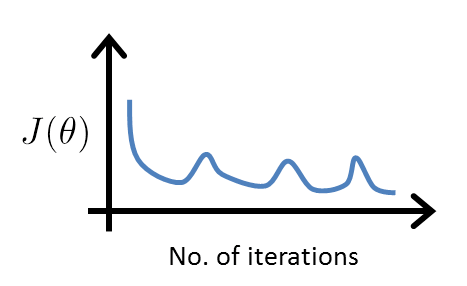### 4. 如何初始化聚类中心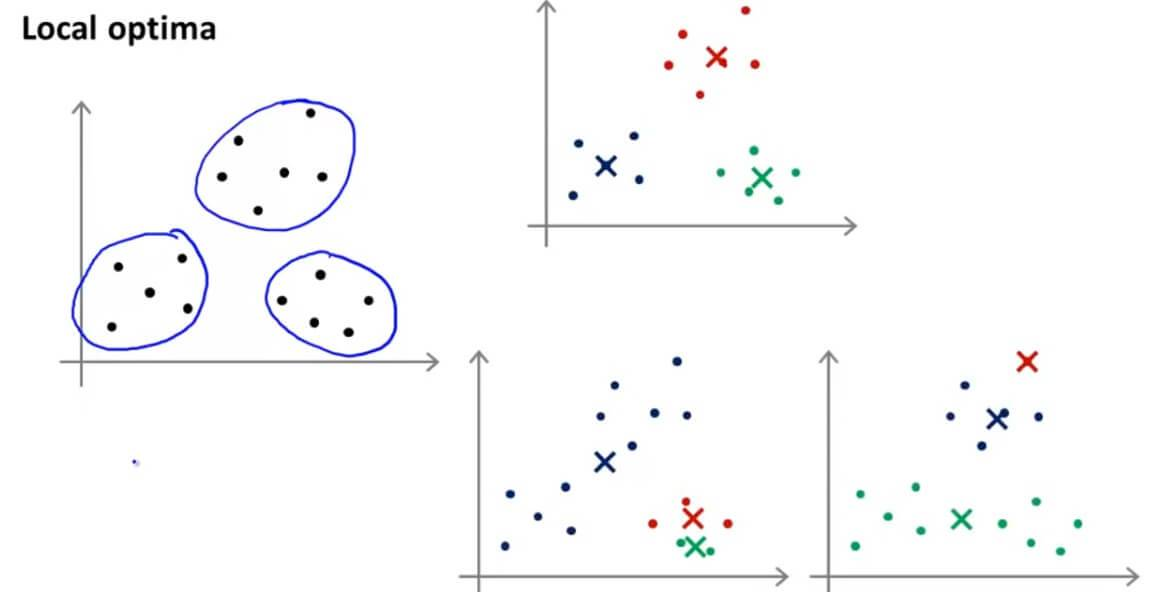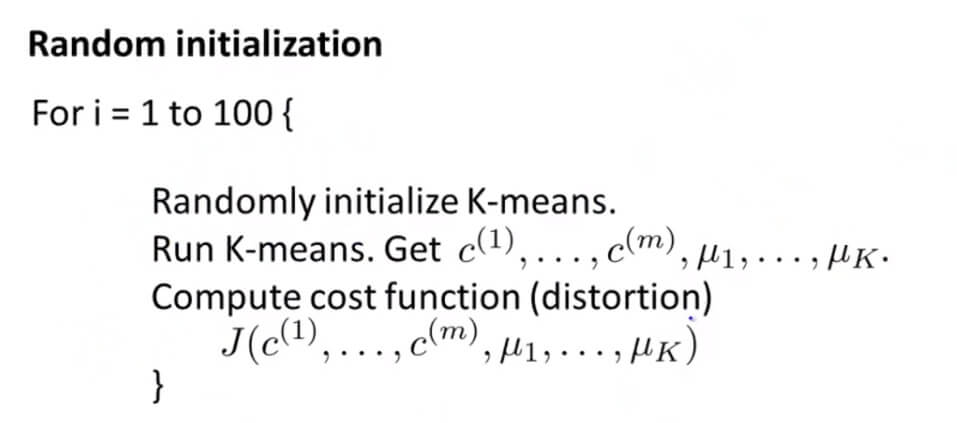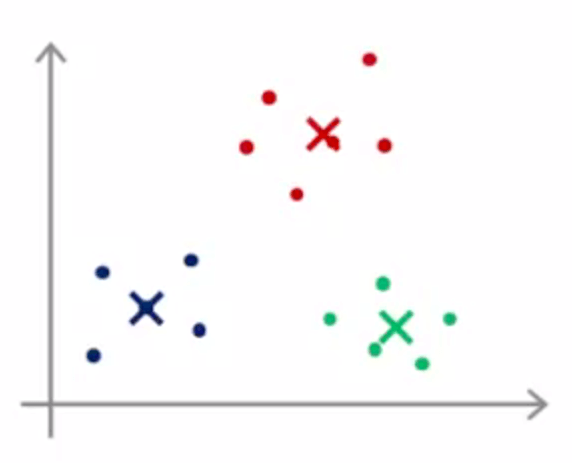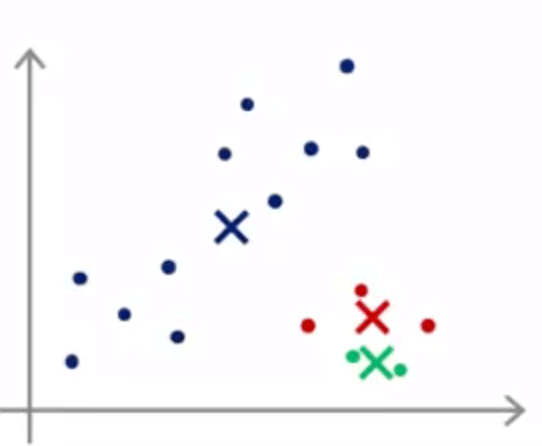for i=1 to 100 (一般循环选择在 50 - 1000 之间)：

1. 随机初始化，执行 K-Means，得到每个所属的簇 $c^{(i)}$ ，以及各聚类的中心位置 $\mu$ :
$$c^{(1)},c^{(2)},\cdots,c^{(m)};\mu_1,\mu_2,\cdots,\mu_k$$
2. 计算失真函数 J

### 5. 如何确定聚类数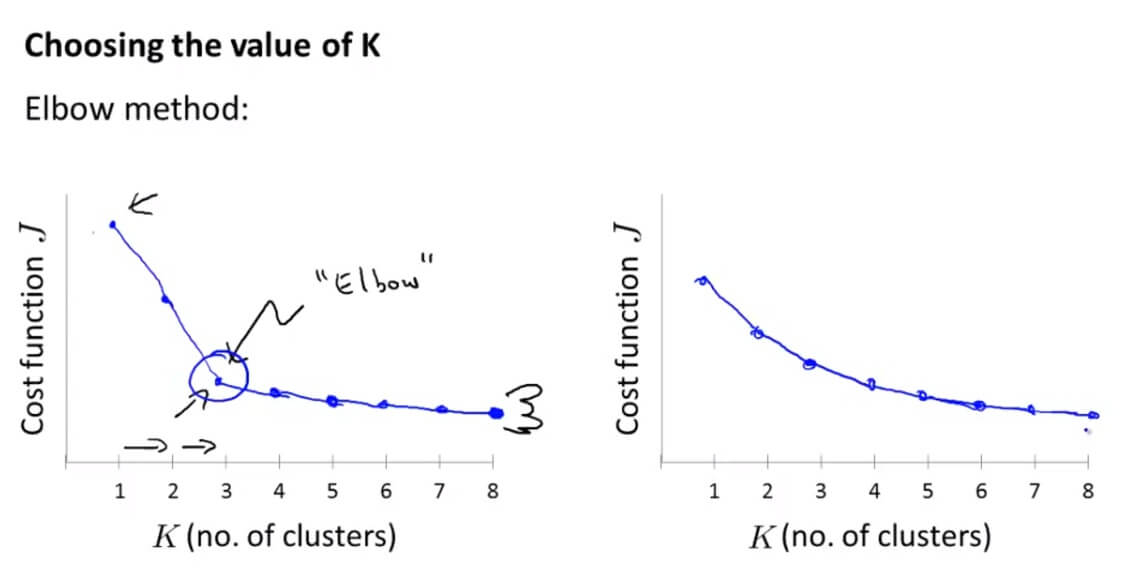## 二. Unsupervised Learning 测试

### 1. Question 1

For which of the following tasks might K-means clustering be a suitable algorithm? Select all that apply.

A. Given a database of information about your users, automatically group them into different market segments.

B. Given sales data from a large number of products in a supermarket, figure out which products tend to form coherent groups (say are frequently purchased together) and thus should be put on the same shelf.

C. Given historical weather records, predict the amount of rainfall tomorrow (this would be a real-valued output)

D. Given sales data from a large number of products in a supermarket, estimate future sales for each of these products.

A.前面的细分市场例子。
B.细分市场的实例。
C.给出历史的天气记录，那也就是说明确知道了真实值。属于监督学习。
D.同C。

### 2. Question 2

Suppose we have three cluster centroids $\mu_{1} = \begin{bmatrix} 1\\ 2 \end{bmatrix}$, $\mu_{2} = \begin{bmatrix} -3\\ 0 \end{bmatrix}$ and $\mu_{3} = \begin{bmatrix} 4\\ 2 \end{bmatrix}$. Furthermore, we have a training example $x^{(i)} = \begin{bmatrix} 3\\ 1 \end{bmatrix}$. After a cluster assignment step, what will $c^{(i)}$ be?

A. $c^{(i)} = 1$

B. $c^{(i)} = 3$

C. $c^{(i)} = 2$

D. $c^{(i)}$ is not assigned

### 3. Question 3

K-means is an iterative algorithm, and two of the following steps are repeatedly carried out in its inner-loop. Which two?

A. Using the elbow method to choose K.

B. The cluster assignment step, where the parameters $c^{(i)}$ are updated.

C. Feature scaling, to ensure each feature is on a comparable scale to the others.

D. Move the cluster centroids, where the centroids $\mu_{k}$ are updated.

### 4. Question 4

Suppose you have an unlabeled dataset $\begin{Bmatrix} x^{(1)},\cdots,x^{(m)} \end{Bmatrix}$. You run K-means with 50 different random initializations, and obtain 50 different clusterings of the data. What is the recommended way for choosing which one of these 50 clusterings to use?

A. The only way to do so is if we also have labels y(i) for our data.

B. For each of the clusterings, compute $\frac{1}{m}\sum^{m}_{i=1}\left \| x^{(i)} - \mu_{c}^{(i)} \right \|^{2}$, and pick the one that minimizes this.

C. Always pick the final (50th) clustering found, since by that time it is more likely to have converged to a good solution.

D. The answer is ambiguous, and there is no good way of choosing.

### 5. Question 5

Which of the following statements are true? Select all that apply.

A. If we are worried about K-means getting stuck in bad local optima, one way to ameliorate (reduce) this problem is if we try using multiple random initializations.

B. Since K-Means is an unsupervised learning algorithm, it cannot overfit the data, and thus it is always better to have as large a number of clusters as is computationally feasible.

C. The standard way of initializing K-means is setting $\mu_{1},\cdots,\mu_{k}$ to be equal to a vector of zeros.

D. For some datasets, the "right" or "correct" value of K (the number of clusters) can be ambiguous, and hard even for a human expert looking carefully at the data to decide.

A. 为了减少陷入局部最优的结果，可以多次选取随机初始参数。
B. 因为非监督学习没有过拟合所以可以选取更多的簇，那显然是不对的，不是越多的簇越好，而是看我们的要求的。
C. 初始化聚类中心应该是让其随机等于训练样本的值。
D. K值很难确定，正确。

GitHub Repo：Halfrost-Field

Follow: halfrost · GitHubPrevious Post

Next Post